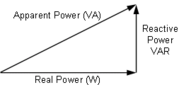## FANDOM

655 Pages

Electric power is the amount of work done by an electric current in a unit time. When a current flows in a circuit with resistance, it does work. Devices can be made that convert this work into:

1. heat (electric heaters), light (light bulbs and neon lamps)
2. motion, that is, kinetic energy (electric motors).

Electric power, like mechanical power, is represented by the letter P in electrical equations, and is measured in units called watts (symbol W), after Scottish engineer James Watt.

## DescriptionEdit

In resistive circuits, instantaneous electrical power is calculated using Joule's Law, which is named for British physicist James Joule, who first showed that electrical and mechanical energy were interchangeable.

$P=IV$

where

P = power in watts
I = current in amperes
V = potential difference in volts

In reactive circuits, energy storage elements such as inductance and capacitance may result in periodic reversals of the direction of energy flow. The portion of power flow that, averaged over a complete cycle of the AC waveform, results in net transfer of energy in one direction is known as real power. That portion of power flow due to stored energy, that returns to the source in each cycle, is known as reactive power.

The unit for reactive power is given the special name VAR, which stands for volt-amperes-reactive. In reactive circuits, the watt unit (symbol W) is generally reserved for the real power component. The vector sum of the real power and the reactive power is called the apparent power. Apparent power is conventionally expressed in volt-amperes (VA) since it is the simple multiple of rms voltage and current.Power triangle

The relationship between real power, reactive power and apparent power can be expressed by representing the quantities as vectors. Real power is represented as a horizontal vector and reactive power is represented as a vertical vector. The apparent power vector is the hypotenuse of a right triangle formed by connecting the real and apparent power vectors. This representation is often called the power triangle. Using the Pythagorean Theorem, the relationship among real, reactive and apparent power is shown to be:

$real\ power (W)^2 + reactive\ power(VAR)^2 = apparent\ power(VA)^2. \,$

### Power factorEdit

The ratio between real power and apparent power in a circuit is called the power factor. Where the waveforms are purely sinusoidal, the power factor is the cosine of the phase angle (φ) between the current and voltage sinusoid waveforms. Equipment data sheets and nameplates often will abbreviate power factor as "$\cos \phi$" for this reason.

Power factor equals unity (1) when the voltage and current are in phase, and is zero when the current leads or lags the voltage by 90 degrees. Power factor must be specified as leading or lagging. For two systems transmitting the same amount of real power, the system with the lower power factor will have higher circulating currents due to energy that returns to the source from energy storage in the load. These higher currents in a practical system may produce higher losses and reduce overall transmission efficiency. A lower power factor circuit will have a higher apparent power and higher losses for the same amount of real power transfer.

Capacitive circuits cause reactive power with the current waveform leading the voltage wave by 90 degrees, while inductive circuits cause reactive power with the current waveform lagging the voltage waveform by 90 degrees. The result of this is that capacitive and inductive circuit elements tend to cancel each other out. By convention, capacitors are said to generate reactive power whilst inductors are said to consume it (this probably comes from the fact that most real-life loads are inductive and so reactive power has to be supplied to them from power factor correction capacitors).

In power transmission and distribution, significant effort is made to control the reactive power flow. This is typically done automatically by switching inductors or capacitor banks in and out, by adjusting generator excitation, and by other means. Electricity retailers may use electricity meters which measure reactive power to financially penalize customers with low power factor loads (especially larger customers).

### Kilowatt-hourEdit

When paired with a unit of time, the term watt is used for expressing energy consumption. For example, a kilowatt hour, is the amount of energy expended by a one kilowatt device over the course of one hour; it equals 3.6 megajoules. A megawatt day (MWd or MW·d) is equal to 86.4 GJ.

These units are often used in the context of power plants and home energy bills. Electricity utilities bill residential customers for only real power consumed, as opposed to apparent power. Industrial customers are more scrutinized as they are penalized for electric loads that have low power factors which create a large difference between the supplied apparent power and the power consumed by the load (real power).

## ApplicationsEdit

There are few places in the world today where electricity is not utilised; be it in industries, office and residential buildings, or public places.

For all these places electricity is supplied by a utility company licensed or authorised for this purpose observing the procedures laid down by law, which takes into account the electric power supply as explained.

## See alsoEdit

 This page uses Creative Commons Licensed content from Wikipedia (view authors).Community content is available under CC-BY-SA unless otherwise noted.